#ver. 9.2

last update; Mar. 13. 2018

## Calculation of gene coexpression data (ver. c4.1)

### Data source

1388 GeneChip data (25k, 46 series) downloaded from TAIR (Microarray data from AtGenExpress).

### Normalization

1. RMA normalization was applied to the 46 series
• We used the `justRMA()` function in R/BioConductor with default options.
2. Some series were divided into sub-series according to genotypes or tissues to be treated;
• ME00325, ME00326, ME00327, ME00328, ME00329, ME00330, ME00338 and ME00340 were divided into shoot and root.
• ME00339 was divided into shoot, root and cell suspension culture.
• ME00335 and ME00343 were divided into wild type and mutant.
3. Genes were normalized by those expression levels in the 58 experimental sub-series.
• For each gene, the average expression value across all chips in the sub-series was subtracted.
4. All sub-series were combined into one gene expression table.

### Calculation of sample redundancy (~ver7, microarray coexpression)

Some data such as a large series of time-course experiments under a single biological condition are biologically redundant or biased. Since these biases may mislead to incorrect conclusions, we have corrected these possible redundancies and biases based on Pearson's correlation coefficients (PCCs) between samples.

1. First, PCCs between sample S1 and sample S2 were calculated.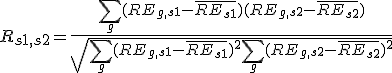, where REg,s is the relative expression of gene G in sample S,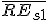is the average relative expression value for all genes in sample S1;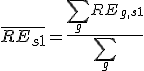,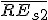is the average relative expression value for all genes in sample S2;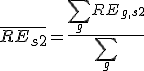.

2. For the paiwise sample redundancy (Js1,s2) between sample S1 and sample S2, we introduced the cut-off threshold C to Rs1,s2.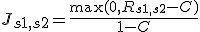.
We used 0.4 for this threshold, which is roughly optimized.

3. The sample redundancy Js1 for sample S1 is calculated as the summation of the pairwise sample redundancies between sample S1 and each of all samples including sample S1 itself.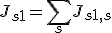4. The weight of sample S1 is the inverse of the square root of the sample redundancy Js1. This procedure is analogous to the calculation of the standard error from the standard deviation. If sample S1 is replicated 4 times with no experimental error, the reliability of the data for sample S1 become double.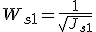### PCC of a pair of gene expression patterns

The weighted PCC (CORg1,g2) was calculated between gene G1 and gene G2.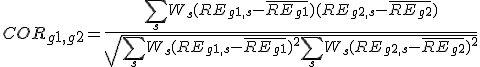, where REg,s is relative expression of gene G in sample S,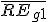is the weighted average relative expression value of gene G1;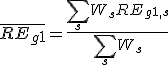,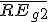is the weighted average relative expression value of gene G2;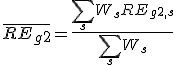.

### Mutual Rank (MR) for gene pairs

From the coexpression version c4.0, we do not use this PCC for the selection of coexpressed genes. This PCC is transformed to MR. (Rank and Mutual Rank)

This page was written on Sep. 16. 2008 for ATTED-II version 5.2.